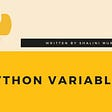# Python range() Function

`for number in range(0,6):    print(number)`
`012345`
`for number in range(0, 60, 10):    print(number)`
`01020304050`
`for number in range(5,0, -1):    print(number)`
`54321`

# Python range() Function in Detail

`for number in range(some_number):    # Do something`
`1. range(end)2. range(start, end)3. range(start, end, stepsize)`

## 1. range(end)—Only Specify the End Value for a Range

`nums = range(6)`
`range(0, 6)`
`nums = list(range(6))print(nums)`
`[0, 1, 2, 3, 4, 5]`

## 2. range(start, end)—Specify Both Start and End Values for a Range

`range(-2,3)`
`rng = range(-2, 3)nums_list = list(rng)print(nums_list)`
`[-2, -1, 0, 1, 2]`

## 3. range(start, end, stepsize)—Range with a Custom Step Size

`rng = range(-20, 30, 10)nums_list = list(rng)print(nums_list)`
`[-20, -10, 0, 10, 20]`

# Python range() Function Usage Examples

## Use range() with a For Loop

`for number in range(0, 6):    print(number)`
`012345`

## Increment with Python’s range() Function

`for num in range(0, 4):    print(num)`
`0123`

## Decrement with Python’s range() Function

`for num in range(4, 0, -1):    print(num)`
`4321`
`for num in reversed(range(1, 5)):    print(num)`
`4321`

## Ignore the Index When Looping

`for i in range(5):    print("Hello!")`
`Hello!Hello!Hello!Hello!Hello!`
`for _ in range(5):    print("Hello!")`
`Hello!Hello!Hello!Hello!Hello!`

# Do Not Use range() Function Blindly

`queue = ["Jack", "Ann", "Sofie"]for i in range(len(queue)):    name = queue[i]    print(f"{name} is at {i+1}. position")`
`Jack is at 1. positionAnn is at 2. positionSofie is at 3. position`
`queue = ["Jack", "Ann", "Sofie"]  for pos, name in enumerate(queue):    print(f"{name} is at {pos + 1}. position")`
`Jack is at 1. positionAnn is at 2. positionSofie is at 3. position`

# Conclusion

• Numbers in increasing order.
• Numbers in decreasing order.
• Numbers with a custom step size.

# References

--

--

--

## More from Artturi Jalli

I’m a tech expert, entrepreneur, and software engineer from Finland. I write about programming, tech, and science. Visit my blog at www.codingem.com.

Love podcasts or audiobooks? Learn on the go with our new app.

## Build Season Week 4 Recap## Are Developers with Python Skills in Switzerland Happy and Well-paid?## Spice you Blends! The Super Blender is here!## How I created a bot that tweets the correct Wordle word daily## Slack System Architecture## Improve and Fix Slow Magento 2 Performance Top issues## Artturi Jalli

I’m a tech expert, entrepreneur, and software engineer from Finland. I write about programming, tech, and science. Visit my blog at www.codingem.com.

## Learning Python - 9 Built-in Functions For Data science## Variables in Python